Forecasting Principles and Practices Load the library fpp2

``library(fpp2)``
``````autoplot(melsyd[,"Economy.Class"])+
ggtitle("Economy class passengers:Melbourne-Syney")+
xlab("Year")+
ylab("Thousands")``````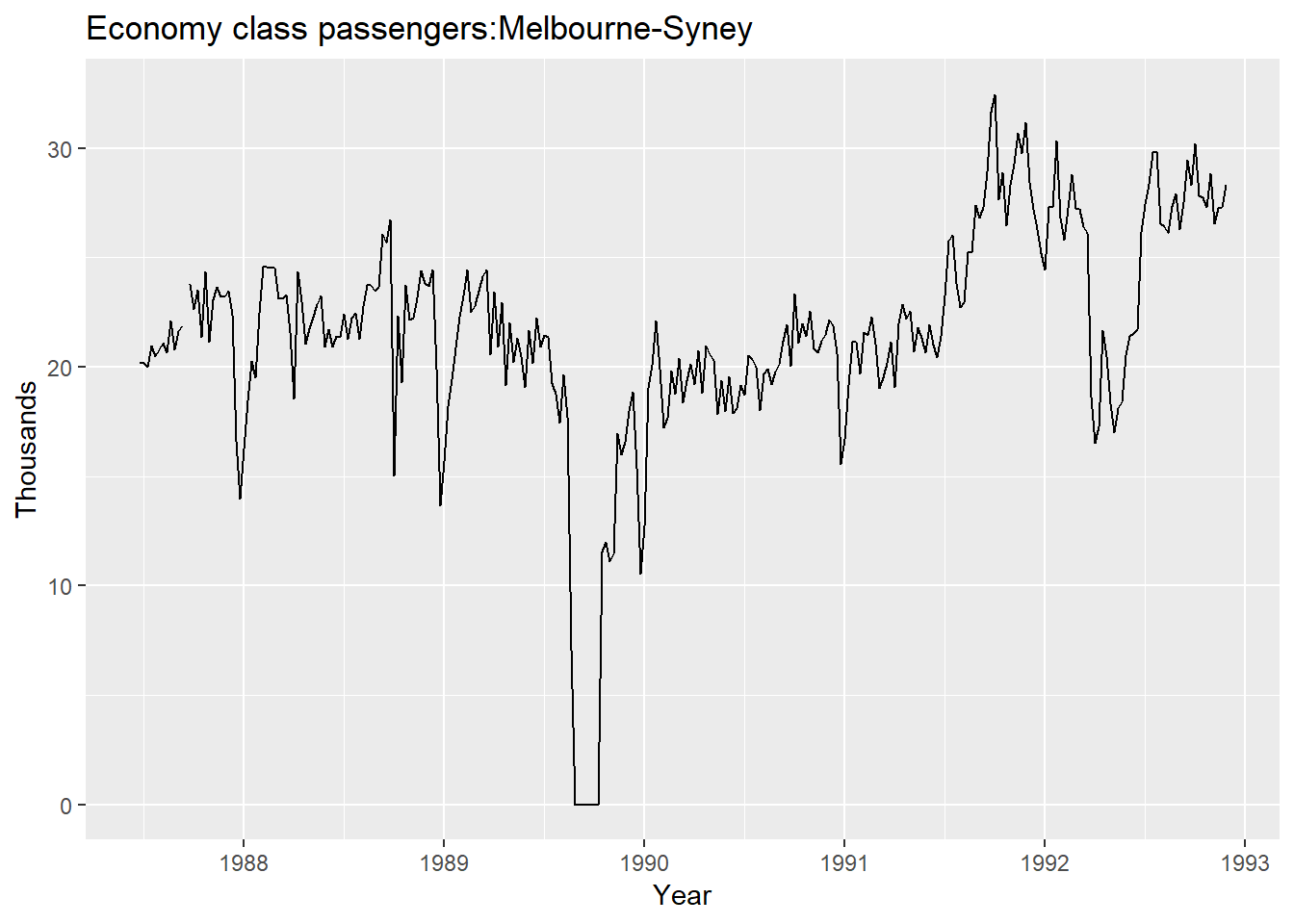## Seasonal Plots

``````ggseasonplot(a10,year.labels = TRUE,year.lables.left=TRUE)+
ylab("\$ million")+
ggtitle("Seasonal plot: antidiabetic drug sales")``````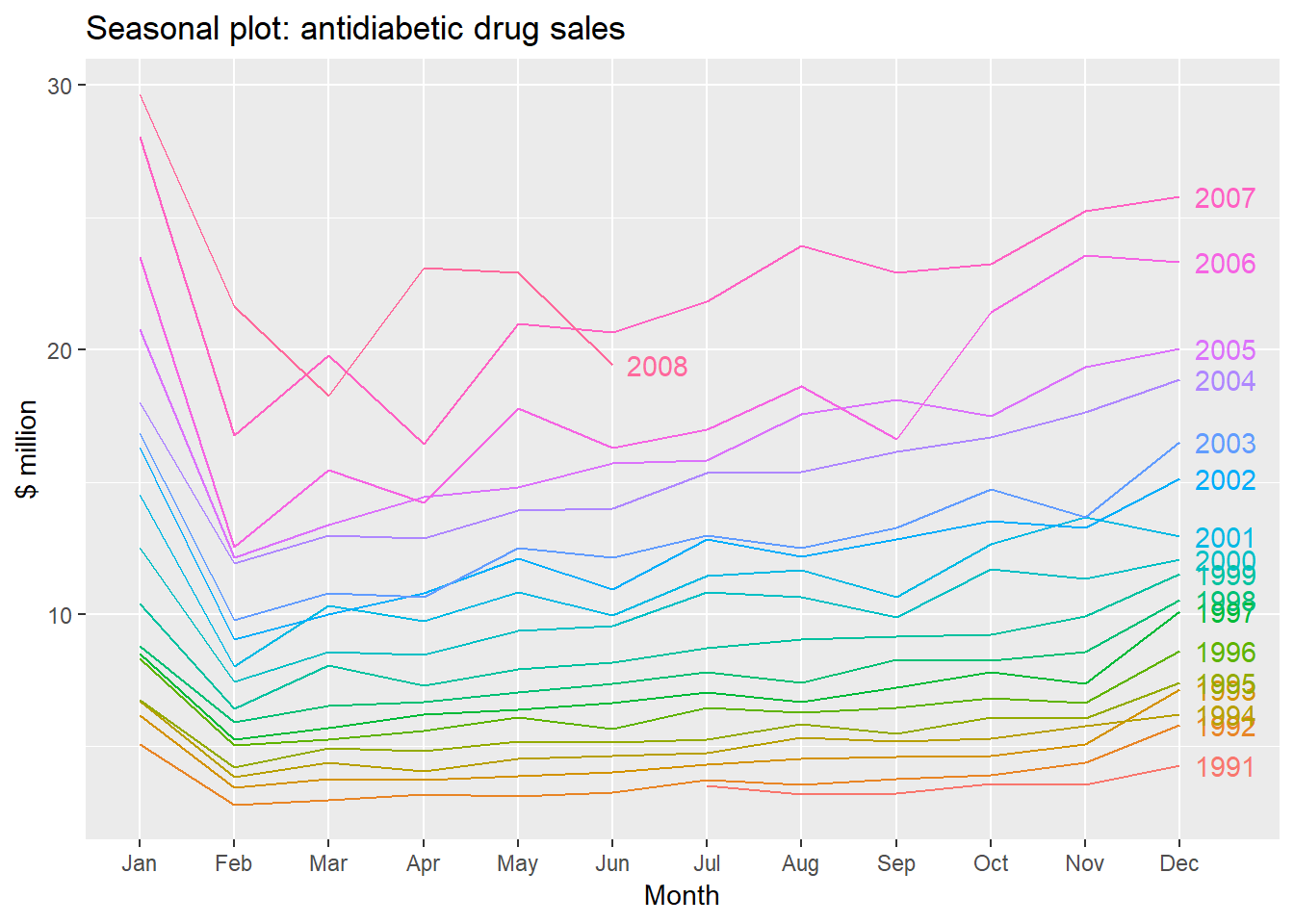You can display it by polar coordinates also

``````ggseasonplot(a10, polar=TRUE) +
ylab("\$ million") +
ggtitle("Polar seasonal plot: antidiabetic drug sales")``````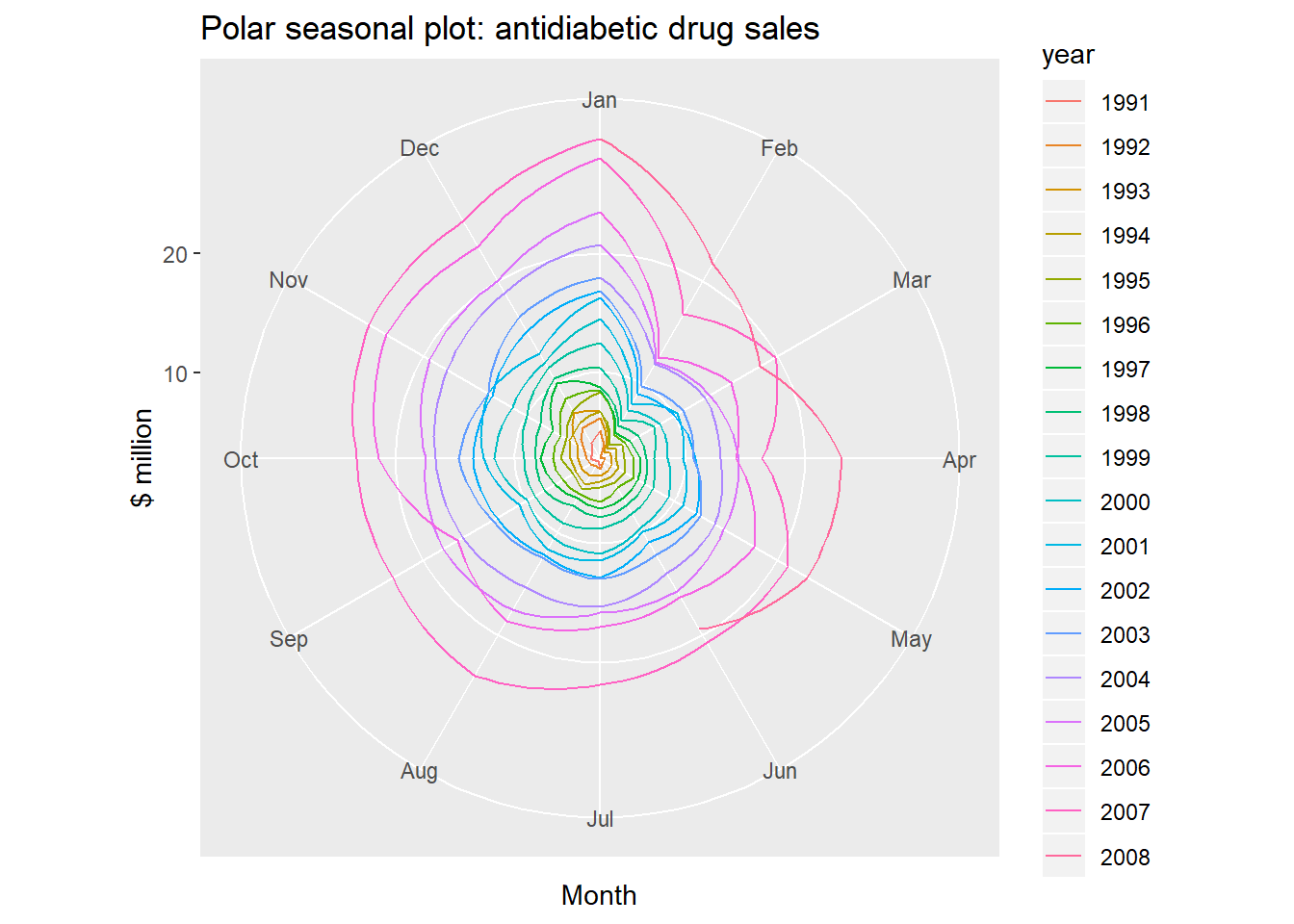Season Subseries Plot: It emphasies the seasonal patterns where the data for each season are collected together in separate mini time phases.

``````ggsubseriesplot(a10) +
ylab("\$ million") +
ggtitle("Seasonal subseries plot: antidiabetic drug sales")``````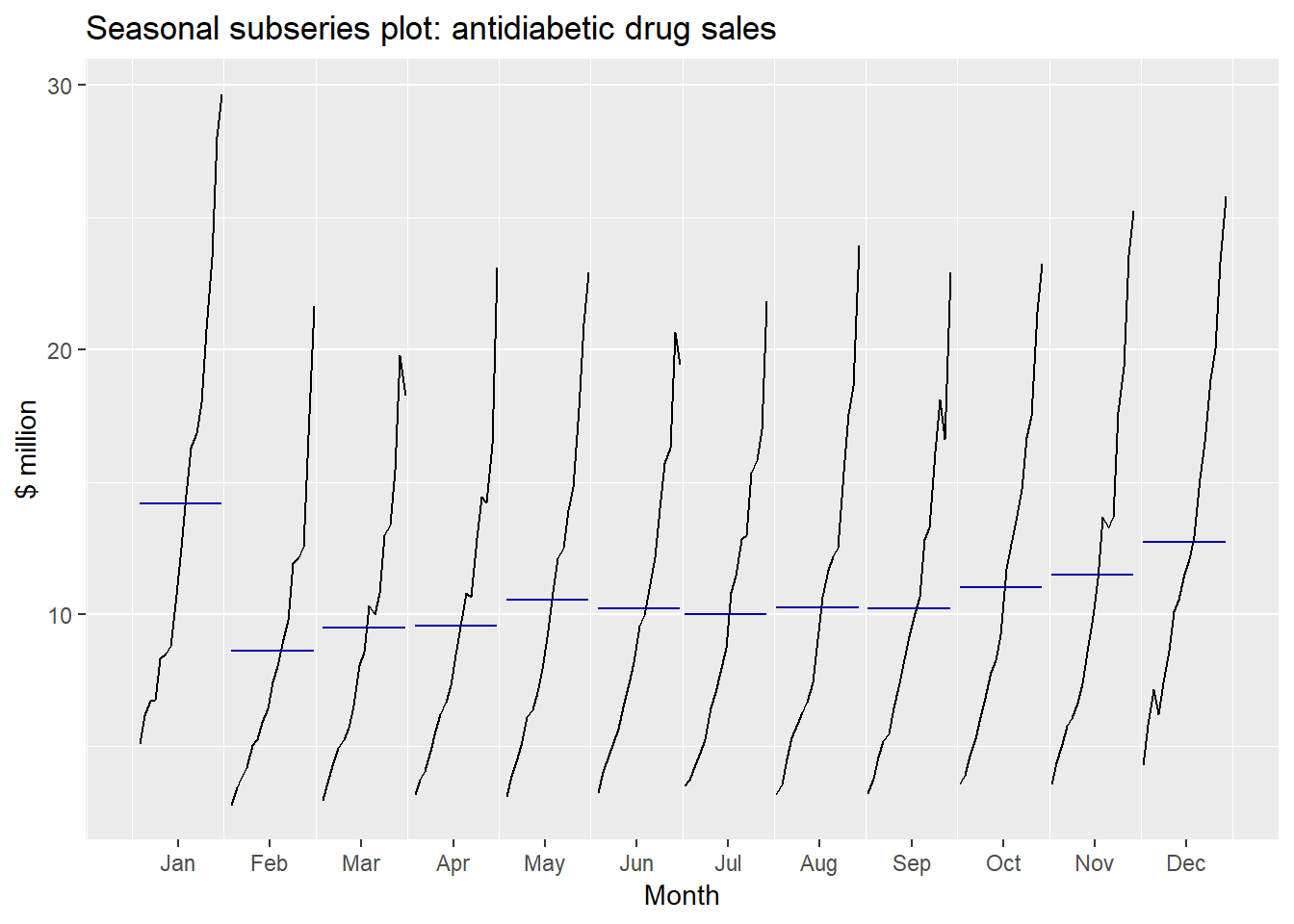The horizontal lines are means for each month. This also indicates the change in seasonality over time.

Scatterplots are used to explore relationship between time series Figure shows two time series: half hourly electricity demand ( in Gigawatts) and temperature ( Gigawatts)

``````autoplot(elecdemand[,c("Demand","Temperature")], facets=TRUE) +
xlab("Year: 2014") + ylab("") +
ggtitle("Half-hourly electricity demand: Victoria, Australia")``````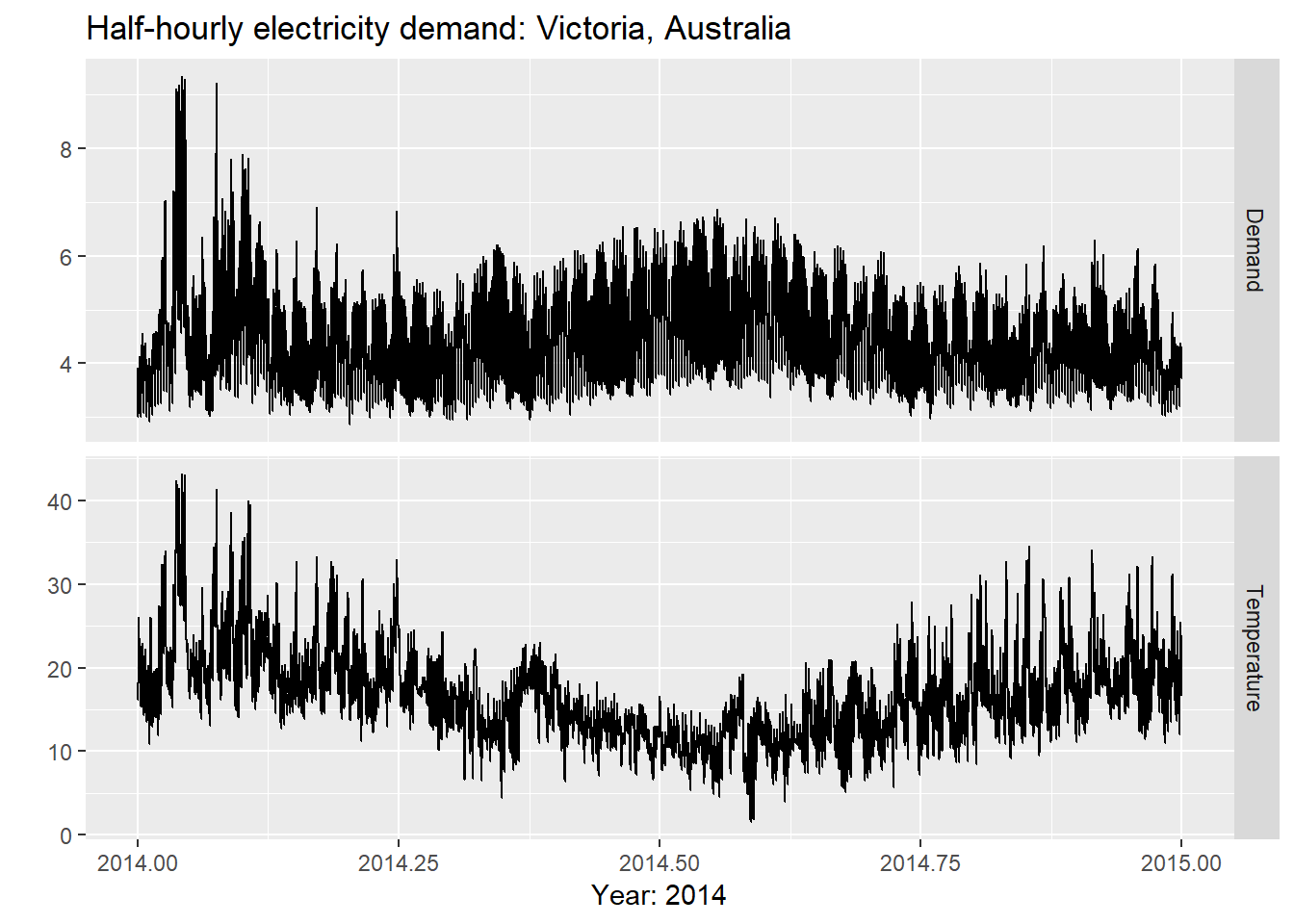We can see the relationship between demand and temperature as below

``````qplot(Temperature, Demand, data=as.data.frame(elecdemand)) +
ylab("Demand (GW)") + xlab("Temperature (Celsius)")``````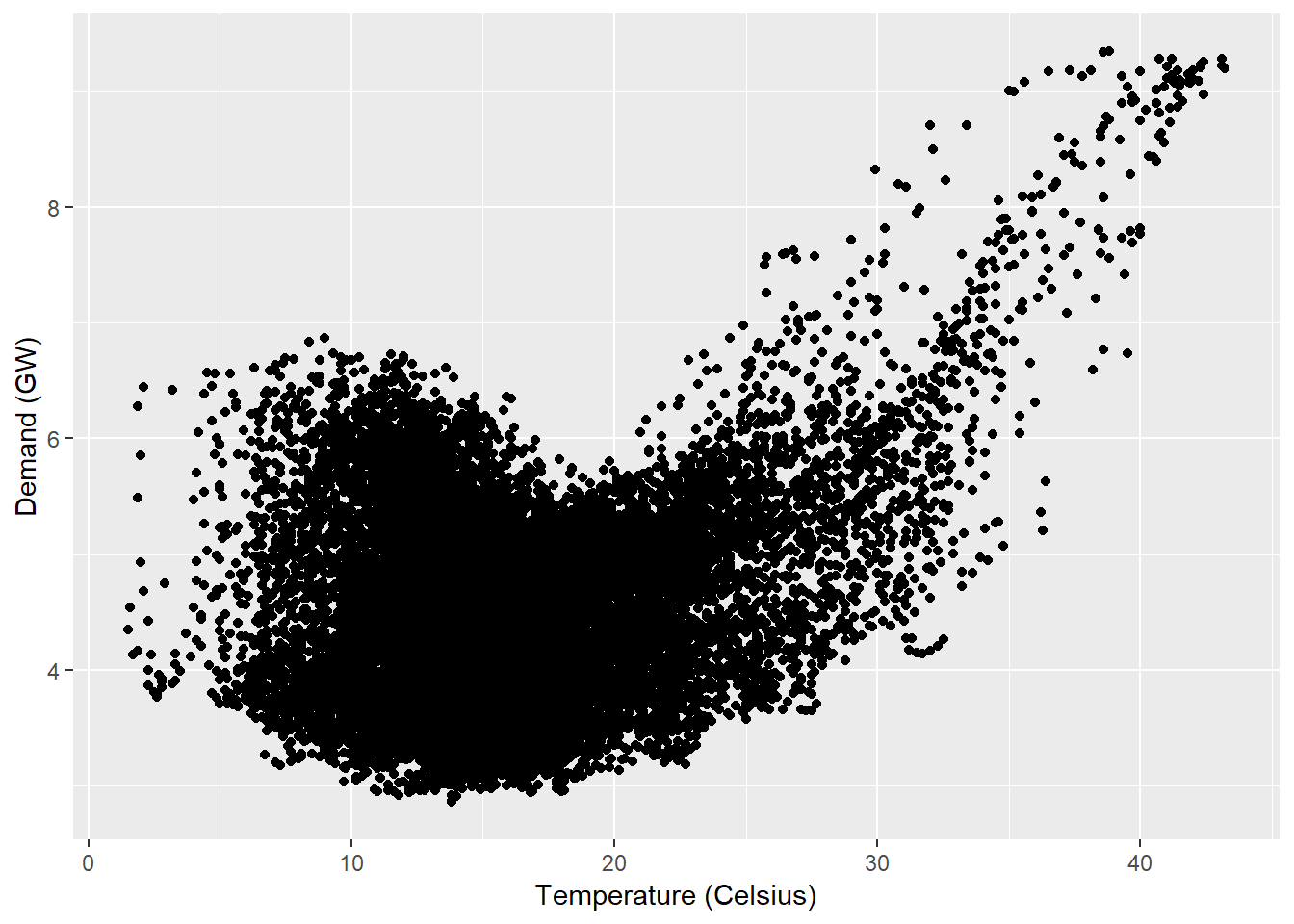Scatterplot matrices Quarterly visitor numbers for five seasons.

``````autoplot(visnights[,1:5], facets=TRUE) +
ylab("Number of visitor nights each quarter (millions)")``````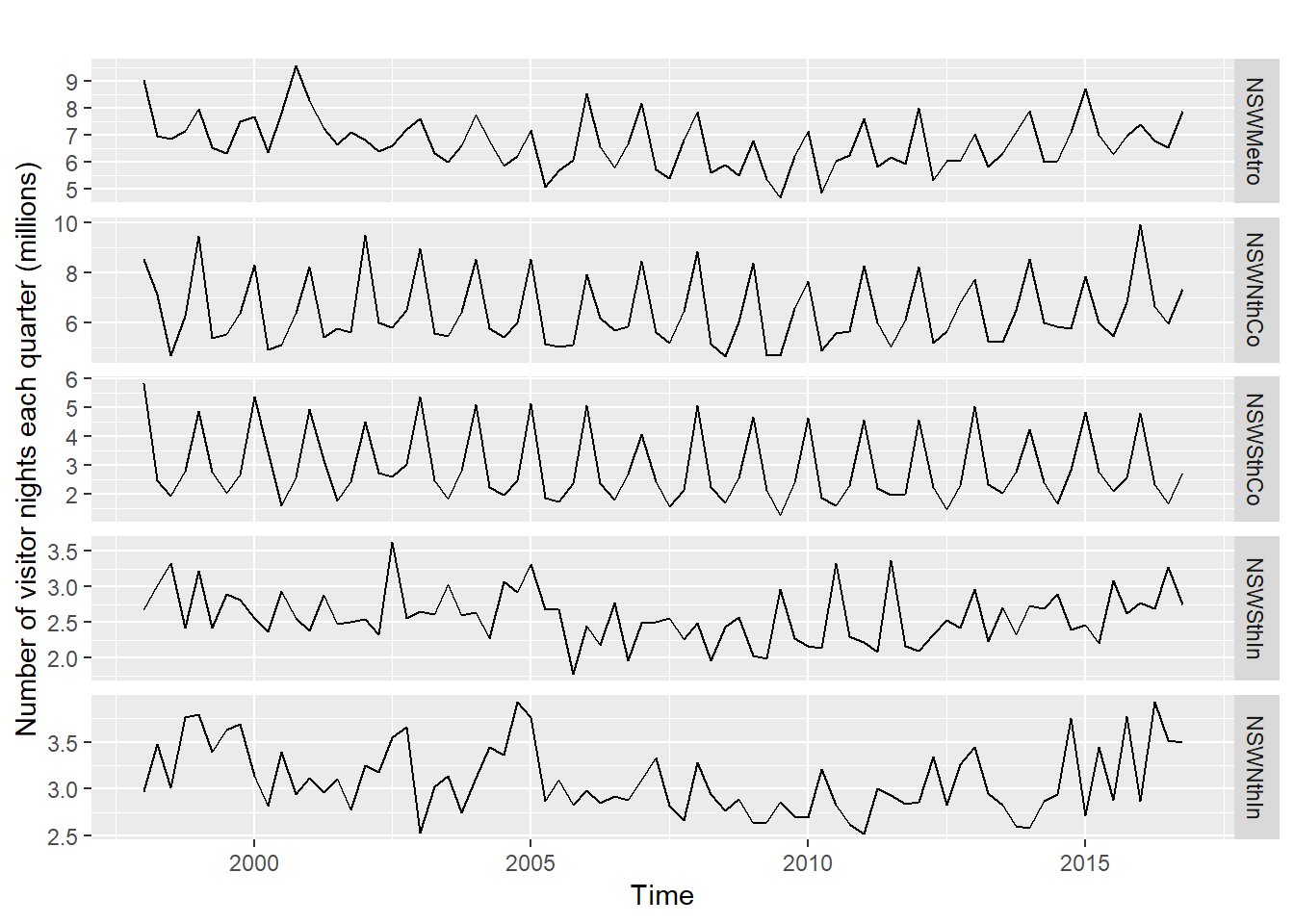To see the scatterplot matrix pairwise

``GGally::ggpairs(as.data.frame(visnights[,1:5]))``
``````## Registered S3 method overwritten by 'GGally':
##   method from
##   +.gg   ggplot2``````